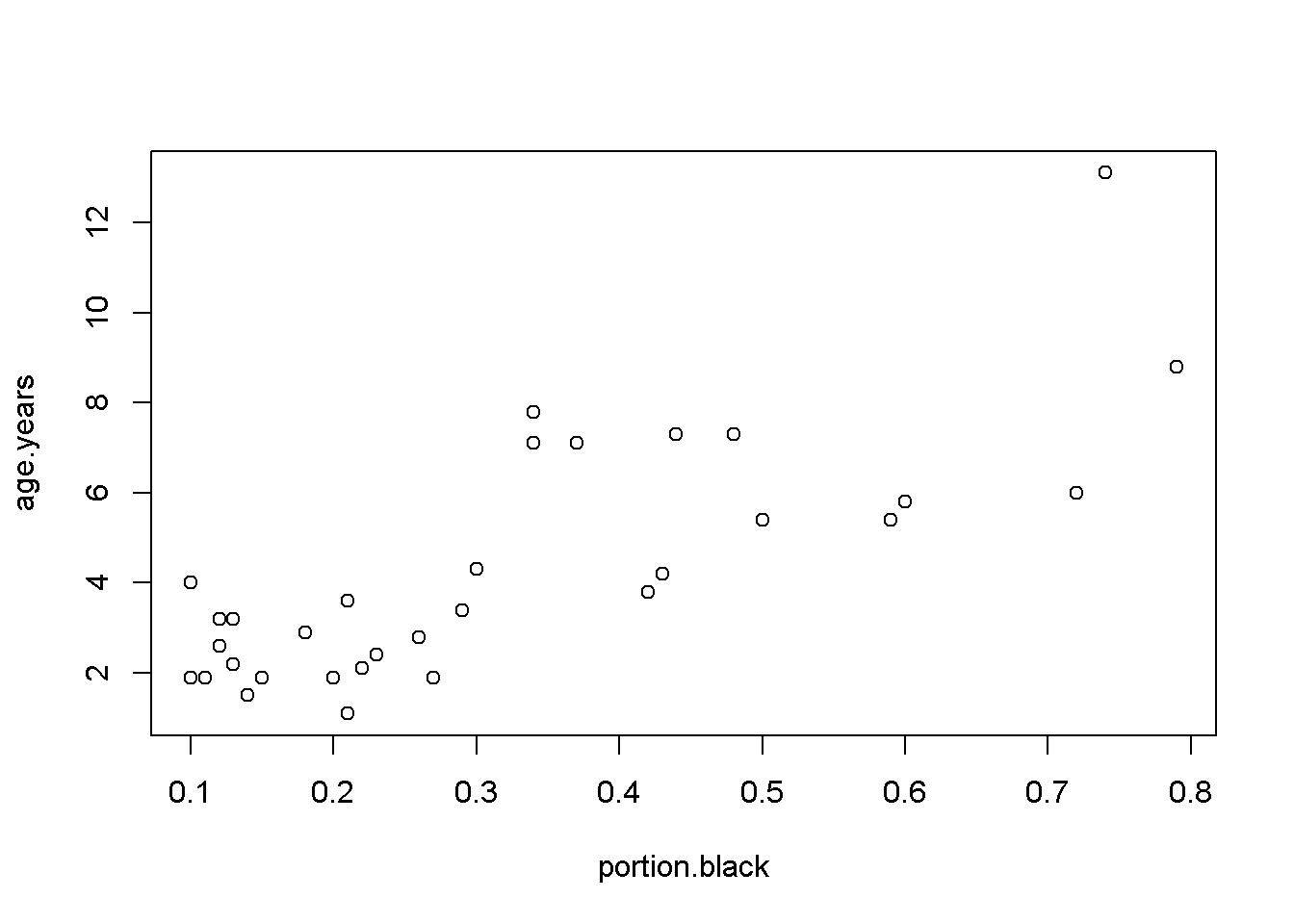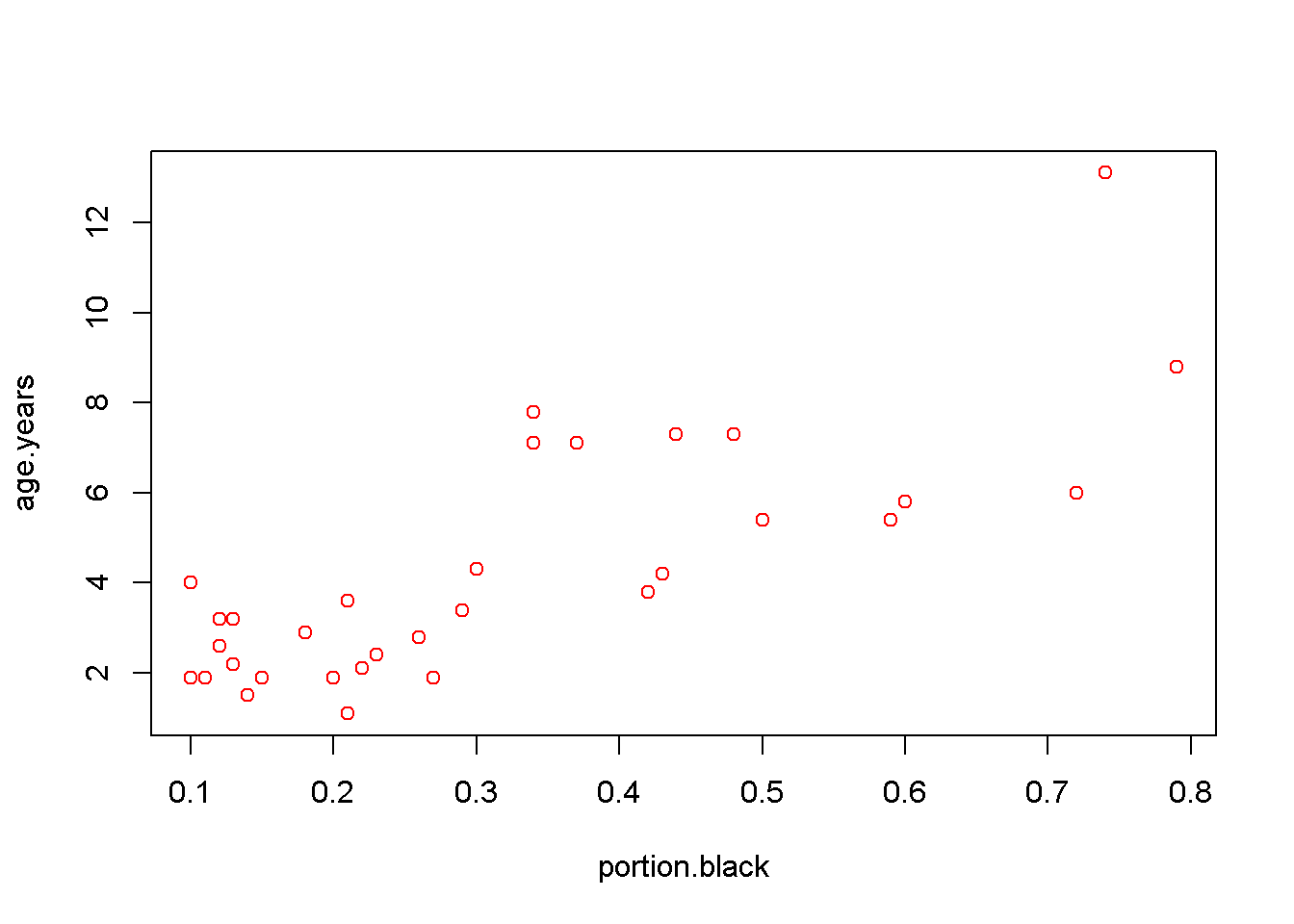1 Introduction

The goal of this lab is to * Review assumptions of ANOVA/t-tests/regression * Review diagnostics plots for normality and constant variance * Introduce diagnostics plots for outliers * Investigate the role of transformation on diagnostic plots * Introduce how to model curved (non-linear) data with x^2 terms * BONUS: material on R^2 for tomorrow’s lecture occurs at the end

Source Data

Data on the relationship between the amount of pigment on lion snouts and their age from Whitman et al (2004). These data are featured in Chapter 17 of Whitlock & Shulter’s Analysis of Biological Data, 2nd ed.

The original data was presented in Figure 4, pg 2, of Whitman

References: Whitman, K, AM Starfield, HS Quadling and C Packer. 2004. Sustainable trophy hunting of African lions. Nature.

1.1 Preliminaries

#The following sets up the data fro the analysis
#Set working directory

setwd("C:/Users/lisanjie2/Desktop/TEACHING/1_STATS_CalU/1_STAT_CalU_2016_by_NLB/Lecture/Unit3_regression/last_week")

2 Plot Raw Data

2.1 Basic R plot

plot(age.years ~ portion.black,
data = dat)2.2 Change color w/ col =

plot(age.years ~ portion.black,
data = dat,
col = 2)<